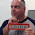## Tuesday, June 28, 2022

### Moving content of data structure into an arrayI have been asked this same question a few times during the last couple of weeks: What is easiest way to move data from a data structure's subfields into an array?

Asking some other people I know how they would do it, several of them did not know of this simple way.

Here is the start of my code:

 ```01 **free 02 dcl-ds DataStructure ; 03 Subfield01 char(10) ; 04 Subfield02 like(Subfield01) ; 05 Subfield03 like(Subfield01) ; 06 Subfield04 like(Subfield01) ; 07 Subfield05 like(Subfield01) ; 08 Subfield06 like(Subfield01) ; 09 Subfield07 like(Subfield01) ; 10 Subfield08 like(Subfield01) ; 11 Subfield09 like(Subfield01) ; 12 Subfield10 like(Subfield01) ; 13 Array like(SubField01) dim(10) samepos(SubField01) ; 14 end-ds ; ```

Line 1: All of my code is always totally free RPG.

Line 2 – 14: This is the data structure I am using for this example.

Line 3: This is the definition of the first subfield, which is defined as character and is ten long.

Lines 4 – 12: I have defined the other subfields using the LIKE keyword so that they will be the same as Subfield01.

Line 13: I have defined an array within the data structure. It has a dimension of ten elements. The SAMEPOS denotes that the array starts at the same position as Subfield1.

If you are using an older release that does not support SAMEPOS you will need to use the following in this line's place:

 ```13 Array like(Subfield01) dim(10) pos(1) ; ```

This line says that the array starts at position 1 of the data structure, i.e., at the start of Subfield01.

The result is that the array elements overlay the subfields.

As the array overlays the data structure subfields I can load the subfields using the %LIST built in function to load the array in one statement.

 ```15 Array = %list('First' : 'Second' : 'Third' : 'Fourth' : 'Fifth' : 'Sixth' : 'Seventh' : 'Eighth' : 'Ninth' : 'Tenth') ; ```

If I debug the data structure after this line I can see the contents of both the array and the subfields:

 ```> EVAL datastructure ARRAY OF DATASTRUCTURE(1) = 'First ' ARRAY OF DATASTRUCTURE(2) = 'Second ' ARRAY OF DATASTRUCTURE(3) = 'Third ' ARRAY OF DATASTRUCTURE(4) = 'Fourth ' ARRAY OF DATASTRUCTURE(5) = 'Fifth ' ARRAY OF DATASTRUCTURE(6) = 'Sixth ' ARRAY OF DATASTRUCTURE(7) = 'Seventh ' ARRAY OF DATASTRUCTURE(8) = 'Eighth ' ARRAY OF DATASTRUCTURE(9) = 'Ninth ' ARRAY OF DATASTRUCTURE(10) = 'Tenth ' SUBFIELD01 OF DATASTRUCTURE = 'First ' SUBFIELD02 OF DATASTRUCTURE = 'Second ' SUBFIELD03 OF DATASTRUCTURE = 'Third ' SUBFIELD04 OF DATASTRUCTURE = 'Fourth ' SUBFIELD05 OF DATASTRUCTURE = 'Fifth ' SUBFIELD06 OF DATASTRUCTURE = 'Sixth ' SUBFIELD07 OF DATASTRUCTURE = 'Seventh ' SUBFIELD08 OF DATASTRUCTURE = 'Eighth ' SUBFIELD09 OF DATASTRUCTURE = 'Ninth ' SUBFIELD10 OF DATASTRUCTURE = 'Tenth ' ```

In the next part I load data into the subfields:

 ```16 Subfield01 = 'No.1' ; 17 Subfield02 = 'No.2' ; 18 Subfield03 = 'No.3' ; 19 Subfield04 = 'No.4' ; 20 Subfield05 = 'No.5' ; 21 Subfield06 = 'No.6' ; 22 Subfield07 = 'No.7' ; 23 Subfield08 = 'No.8' ; 24 Subfield09 = 'No.9' ; 25 Subfield10 = 'No.10' ; 26 *inlr = *on ; ```

In debug I can check the contents of the data structure at line 26:

 ```> EVAL datastructure ARRAY OF DATASTRUCTURE(1) = 'No.1 ' ARRAY OF DATASTRUCTURE(2) = 'No.2 ' ARRAY OF DATASTRUCTURE(3) = 'No.3 ' ARRAY OF DATASTRUCTURE(4) = 'No.4 ' ARRAY OF DATASTRUCTURE(5) = 'No.5 ' ARRAY OF DATASTRUCTURE(6) = 'No.6 ' ARRAY OF DATASTRUCTURE(7) = 'No.7 ' ARRAY OF DATASTRUCTURE(8) = 'No.8 ' ARRAY OF DATASTRUCTURE(9) = 'No.9 ' ARRAY OF DATASTRUCTURE(10) = 'No.10 ' SUBFIELD01 OF DATASTRUCTURE = 'No.1 ' SUBFIELD02 OF DATASTRUCTURE = 'No.2 ' SUBFIELD03 OF DATASTRUCTURE = 'No.3 ' SUBFIELD04 OF DATASTRUCTURE = 'No.4 ' SUBFIELD05 OF DATASTRUCTURE = 'No.5 ' SUBFIELD06 OF DATASTRUCTURE = 'No.6 ' SUBFIELD07 OF DATASTRUCTURE = 'No.7 ' SUBFIELD08 OF DATASTRUCTURE = 'No.8 ' SUBFIELD09 OF DATASTRUCTURE = 'No.9 ' SUBFIELD10 OF DATASTRUCTURE = 'No.10 ' ```

I can check the contents of the array directly too:

 ```> EVAL array ARRAY OF DATASTRUCTURE(1) = 'No.1 ' ARRAY OF DATASTRUCTURE(2) = 'No.2 ' ARRAY OF DATASTRUCTURE(3) = 'No.3 ' ARRAY OF DATASTRUCTURE(4) = 'No.4 ' ARRAY OF DATASTRUCTURE(5) = 'No.5 ' ARRAY OF DATASTRUCTURE(6) = 'No.6 ' ARRAY OF DATASTRUCTURE(7) = 'No.7 ' ARRAY OF DATASTRUCTURE(8) = 'No.8 ' ARRAY OF DATASTRUCTURE(9) = 'No.9 ' ARRAY OF DATASTRUCTURE(10) = 'No.10 ' ```

Yes, it is that easy to do.

This article was written for IBM i 7.5, and should work for some earlier releases too.

1.Love this

2.Good Information, Love it 😊

3.you can also use good old INZ for the members so you have all in the definition without additional computation.
Exercise for you: can you do the same for an array of DS? How would one initialize them?

1.Use the CLEAR operation code.

4.Excelent Simon thanks for sharing 👍

5.A trick I have been using for years. And if the fields comes from a file you don't have to specify CHAR(10) and LIKE(Subfield01). Instead of naming the overlayed part ARRAY I normally gives it the name of the overlayed fields without the number. In this example I would call it Subfield. It makes it simpler to identify what the field represents. For example Subfield(x) = 1234 is easier to read than array(x) = 1234.

6.Excelente, muy bueno. Gracias Simon.

To prevent "comment spam" all comments are moderated.
```